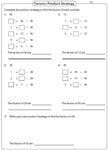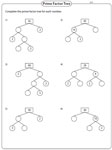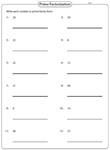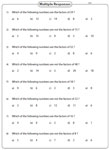# Factors Worksheets

Unique factoring worksheets are available for grade 5 through high school. List out the factors, complete the prime factor tree, draw your own prime factor tree, find the GCF and LCM and explore more worksheets on this page.

Listing out the factors

Knowing how to list out the factors is fundamental to work on the following worksheets.Easy:

Moderate:

Difficult:

Product strategy

Complete the product strategy for each problem and find the factors of the number. Space is also given to write your own product strategy.Easy:

Moderate:

Complete the prime factor Tree

Find the missing prime factors of the number and complete the prime factor tree. The easy level has numbers up to 50 and the moderate level has numbers up to 100.Easy:

Moderate:

Draw your own prime factor tree

Draw your own prime factor tree for each number with the help of branching factors.Easy:

Moderate:

Prime factorization

Prime factorization or prime decomposition is to find the factors, which multiply together to give the original number.Easy:

Moderate:

Difficult:

Multiple responses

Each factoring worksheet has eight questions. Select multiple responses from each objective question.Easy:

Moderate:

Multiple choice questions

Multiple-choice questions are based on finding the factors in the worksheets. The easy level restricts numbers up to 50. The moderate level has up to 100.Easy:

Moderate:

GCF worksheets

Greatest common factors of whole numbers and polynomials are emphasized on this page. Levels from easy to difficult are discussed.

Greatest Common Factor Worksheets

LCM worksheets

A huge collection of least common multiple worksheets is available on this page. Find the LCM of whole numbers and polynomials.

Least Common Multiple Worksheets

Factoring polynomial worksheets

Ample number of worksheets are provided on this page for factoring monomial, binomial and polynomial expressions.

Factoring Polynomial Worksheets

The entire collection of worksheets on factors can be downloaded in a jiffy!

Related Worksheets